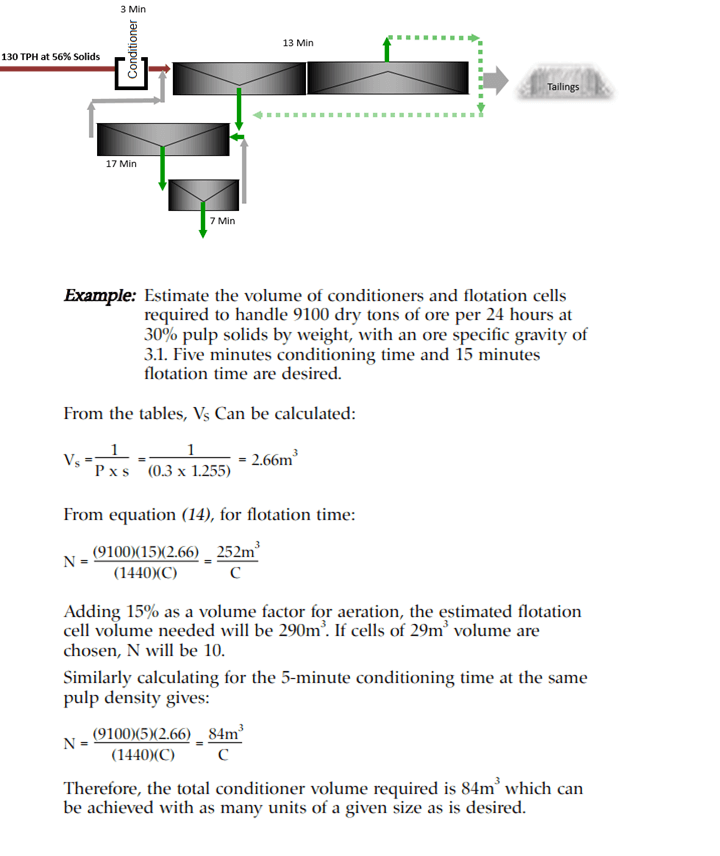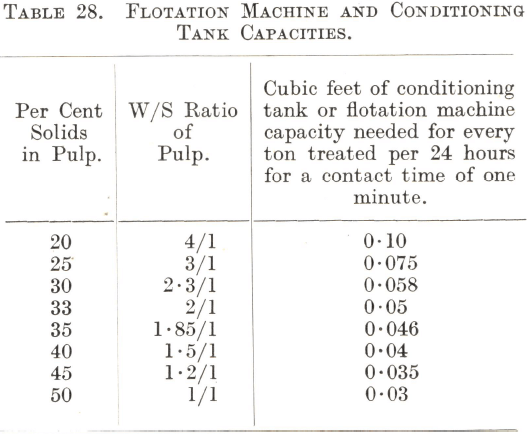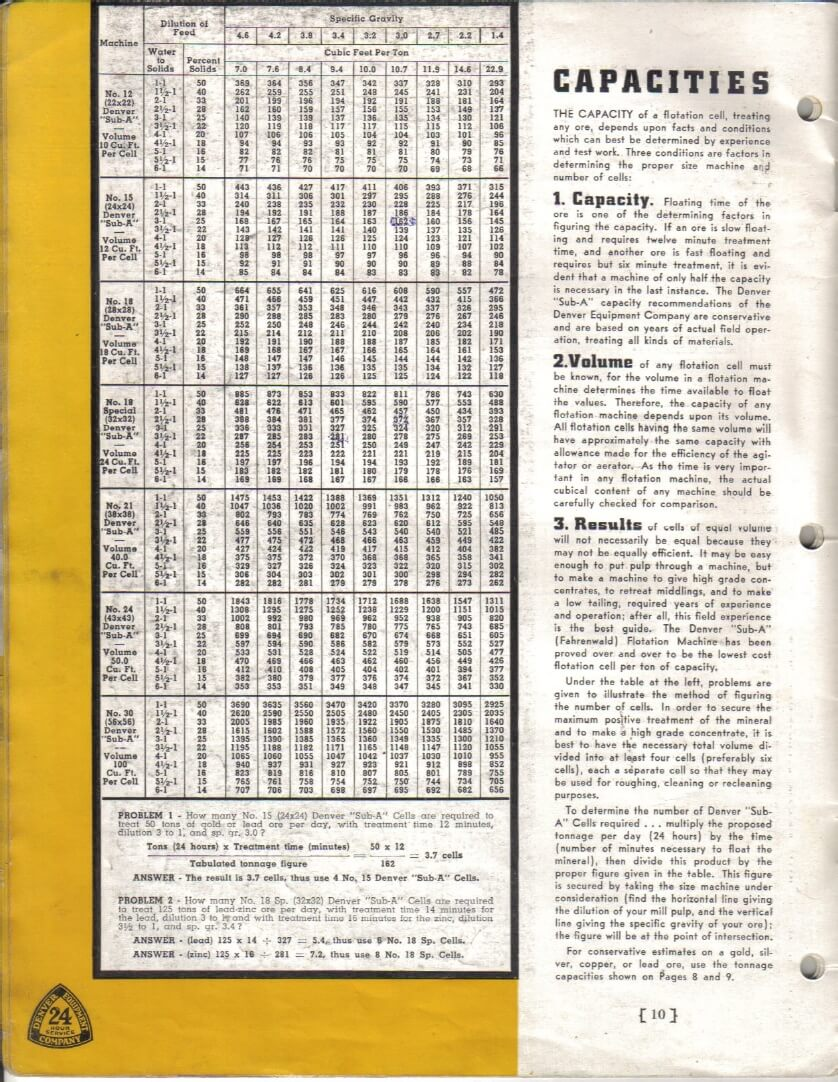# Calculate Flotation Cell Capacities Volume vs Retention Time

This online calculator helps you on how to calculate or estimate either what size/volume flotation cell and well as conditioning tank + how many flotation cells your bank needs to accommodate the retention time you need.  Design Retention time is usually obtained from laboratory testwork and proper use of Lab-to-Plant flotation scale-up factors.

An Example of flotation cell capacity and circuit retention time approximation:
Estimate the volume of conditioners and flotation cells required to handle 9100 dry tons of ore per 24 hours at 30% pulp solids by weight, with an ore specific gravity of 3.1. Five minutes conditioning time and 15 minutes flotation time are desired.
Adding 15% as a volume factor for aeration, the estimated flotation cell volume needed will be 290 cubic meters. If cells of 29,000 Liters volume are chosen, N will be 10.  Similarly calculating for the 5-minute conditioning time at the same pulp density gives (see below).
Therefore, the total conditioner volume required is 84 meters cube which can be achieved with as many units of a given size as is desired.

To determine the minimum number of flotation cells, start with the concept of plug-flow reactors.  This is why some engineers prefer bank of floatation cells while others prefer single cells.  Multiple cells are best performers and achieve better kinetics.Cytec Handbook

Capacity. Floating time of the ore is one of the determining factors in figuring the capacity. If an ore is slow floating and requires twelve minutes treatment time, and another ore is fast floating and requires but six minutes treatment, it is evident that a machine of only half the capacity is necessary in the last instance. The capacity recommendations are conservative and are based on years of actual field operation treating all kind of materials.

Volume of any flotation cell must be known for the volume in a flotation machine determines the time available to float the valves. Therefore, the capacity of any flotation machine depends upon its volume. All flotation cells having the same volume will have approximately the same capacity with allowance made for the efficiency of the agitator or aerator. As the time is very important in any flotation machine, the actual cubical content of any machine should be carefully checked for comparison.

Results of cells of equal volume will not necessarily be equal because they may not be equally efficient. It may be easy enough to put pulp through a machine, but to make a machine to give high grade concentrates, to retreat middlings, and to make a low tailing, required years of experience and operation: after all, this field experience is the best guide.

Under the table at the left, problems are given to illustrate the method of figuring the number of cells. In order to secure the maximum positive treatment of the mineral and to make a high grade concentrate, it is best to have the necessary total volume divided into at least four cells (preferably six cells) each a separate cell so that they may be used for roughing, cleaning or re-cleaning purposes.

To determine the number of Cells required multiply the proposed tonnage per day (24 hours) by the time (number of minutes necessary to float the mineral), then divide this product by the proper figure given in the table. This figure is secured by talking the size machine under consideration (find the horizontal line giving the dilution of your mill pulp, and the vertical line giving the specific gravity of your ore): the figure will be at the point of intersection.

## How to Calculate the Size of Conditioning Tanks & Flotation Machines

If the time needed to condition and float a particular ore is known, the size of the conditioning tank and the number of flotation cells required can be calculated from Table 28.

To find the conditioning tank capacity, the figure in the third column of the table corresponding to the required pulp dilution must be multiplied by the tonnage to be treated per 24 hours and by the contact time in minutes. This will give the size of tank required in cubic feet. It is usual to allow 10 to 20% additional capacity.

To find the flotation machine capacity, the figure in the third column corresponding to the required pulp dilution must be multiplied by the tonnage to be treated per 24 hours and by the time required for flotation in minutes. The product is the number of cubic feet of machine capacity needed. This figure, divided by the capacity in cubic feet of any particular type of flotation cell, will give the number of such cells needed. In the case of the Southwestern Air-Lift Machine it must be divided by 9.85 (the volume in cubic feet of one foot of machine), which will give the length of machine required in feet.A certain amount of discretion must be exercised in making these calculations, particularly when applying laboratory results to plant design. For instance, many types of laboratory flotation machines can be run much harder than is possible in a full-size machine with the result that the time of treatment may be considerably underestimated. Again, the speed of flotation varies in every type of machine. Given an ore which can be successfully floated in a pneumatic cell, the time of treatment may only be 2 to 3 minutes, whereas 10 to 12 minutes or even more may be required in an air-lift machine under exactly similar conditions. Conditioning and flotation contact times obtained in laboratory machines are best checked under pilot plant conditions in machines of the same type that are to be installed in the actual plant.

In selecting the size of a flotation machine by the method described above, it should be remembered that a large cell is more economical in power than a small one. In the absence of specific data, a time of treatment of 12 to 15 minutes is the normal allowance for the flotation of a straightforward ore in a mechanically-agitated or air-lift machine with a W/S ratio between 3/1 and 4/1. If cleaning of the concentrate is likely to be necessary, extra cells will be required, the number varying from one quarter of the number of roughing cells in the case of a low-grade ore to one-half of the roughing cells for one of high grade. When cleaning is practised, it is best to allow for an overall W/S ratio of 4/1, since the water needed for the operation is generally returned to the roughing circuit with consequent dilution of the pulp there. As a rule only the presence of very easily floated mineral in a thick pulp will enable flotation to be accomplished in less than 12 minutes. For example, when a heavily mineralized ore containing lead and zinc sulphides is being treated, it is not uncommon for the pulp to enter the first or lead flotation stage at a W/S ratio of less than 2/1 ; as galena is easily floatable, the time of treatment in this stage is often only 5 to 8 minutes.